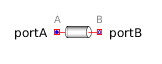Discrete Transfer Function - MapleSim Help

Discrete Transfer Function

Discrete Transfer Function blockDescription The Discrete Transfer Function (or Transfer Function) component defines the transfer function between the input signal $u$ and the output signal $y$. $\frac{y\left(z\right)}{u\left(z\right)}=\frac{{b}_{1}{z}^{{n}_{b}-1}+{b}_{2}{z}^{{n}_{b}-2}+\cdots +{b}_{{n}_{b}}}{{a}_{1}{z}^{{n}_{a}-1}+{a}_{2}{z}^{{n}_{a}-2}+\cdots +{a}_{{n}_{a}}}$ State variables, $x$, are defined according to controller canonical form. Initial values of the states can be set as start values of $x$.Connections

 Name Description Modelica ID $u$ Continuous input signal u $y$ Continuous output signal yParameters

 Name Default Units Description Modelica ID b $\left[1\right]$ Numerator coefficients of transfer function b a $\left[1\right]$ Denominator coefficients of transfer function a Sample Period $0.1$ $s$ Sample period of component samplePeriod ${T}_{0}$ $0$ $s$ First sample time instant startTimeModelica Standard Library The component described in this topic is from the Modelica Standard Library. To view the original documentation, which includes author and copyright information, click here.